• 用户价值分析 RFM模型】用户价值分析
千次阅读
2018-06-11 18:11:00

RFM模型是衡量客户价值和客户创利能力的重要工具和手段。RFM分析模型主要由三个指标组成，下面对这三个指标的定义和作用做下简单解释：

1、最近一次消费(Recency)
最近一次消费意指用户上一次购买的时间，理论上，上一次消费时间越近的顾客应该是比较好的顾客，对提供即时的商品或是服务也最有可能会有反应。因为最近一次消费指标定义的是一个时间段，并且与当前时间相关，因此是一直在变动的。最近一次消费对营销来说是一个重要指标，涉及吸引客户，保持客户，并赢得客户的忠诚度。
2、消费频率(Frequency)
消费频率是顾客在一定时间段内的消费次数。最常购买的消费者，忠诚度也就最高，增加顾客购买的次数意味着从竞争对手处偷取市场占有率，由别人的手中赚取营业额。
3、消费金额(Monetary)
消费金额是对购彩产能的最直接的衡量指标，也可以验证“帕雷托法则”(Pareto’s Law)——公司80％的收入来自20％的顾客。

FRM就是根据客户活跃度和交易金额贡献，进行客户价值细分的一种方法。

RFM算法步骤：
1.计算RFM各项分值
R_S,距离当前日期越近，得分越高，最高7分，最低1分，按实际数据分布情况切割依次从高到低取分数。
F_S,交易频率越高，得分越高，最高7分，最低1分，按实际数据分布情况切割依次从高到低取分数。
M_S,交易金额越高，得分越高，最高7分，最低1分，按实际数据分布情况切割依次从高到低取分数。

2.归总RFM分值
RFM赋予权重（目前权重采用R:F:M = 1:1:1），权重乘以分数归总RFM分值。这个总RFM分值作为衡量用户价值的关键指标。公式如下：

3.根据RFM分值对客户分类

# encoding: utf-8

"""
function：RFM用户价值分析分成5类
author:dongli
update_date:2018-06-07
"""

# 导入包
import pandas as pd
######################################################写入excel设置问题#########################################
import xlsxwriter
# 定义RFM函数
def RFM(aggData):

"""

:param aggData: 输入数据集，数据集字段要包含recency,frequency,monetary等三个字段
:return:返回数据集结果
"""
# 计算R_S
bins = aggData.recency.quantile(q=[0, 0.28, 0.38, 0.46, 0.53, 0.57, 0.77, 1], interpolation='nearest')
bins = 0
labels = [7, 6, 5, 4, 3, 2, 1]
R_S = pd.cut(aggData.recency, bins, labels=labels)

# 计算F_S
bins = aggData.frequency.quantile(q=[0, 0.29, 0.45, 0.60, 0.71, 0.76, 0.90, 1], interpolation='nearest')
bins = 0
labels = [1, 2, 3, 4, 5, 6, 7]
F_S = pd.cut(aggData.frequency, bins, labels=labels)

# 计算M_S
bins = aggData.monetary.quantile(q=[0, 0.20, 0.26, 0.45, 0.55, 0.76, 0.85, 1], interpolation='nearest')
bins = 0
labels = [1, 2, 3, 4, 5, 6, 7]
M_S = pd.cut(aggData.monetary, bins, labels=labels)

# 赋值
aggData['R_S'] = R_S
aggData['F_S'] = F_S
aggData['M_S'] = M_S

# 计算FRM值
aggData['RFM'] = R_S.astype(int)*1 + F_S.astype(int)*1 + M_S.astype(int)*1

# 根据RFM分值对客户分类

#分五类
bins = aggData.RFM.quantile(q=[0, 0.2, 0.4, 0.6, 0.8, 1],interpolation='nearest')
bins = 0
labels = [1, 2, 3, 4, 5]
aggData['level'] = pd.cut(aggData.RFM,bins, labels=labels)

# 分八类
# bins = aggData.RFM.quantile(q=[0, 0.125, 0.25, 0.375, 0.5,0.625, 0.75, 0.875, 1],interpolation='nearest')
# bins = 0
# labels = [1, 2, 3, 4, 5, 6, 7, 8]
# aggData['level'] = pd.cut(aggData.RFM,bins, labels=labels )

return aggData

# 主函数
if __name__ == '__main__':

# 读取数据

# 调用模型函数
result=RFM(aggData)

# 打印结果
print(result)

# 计算每个类别的数据量

c1=list(result["level"].value_counts())

# 计算每个类别所占的百分比

c2 = list(result["level"].value_counts()/len(result)*100)

c3=(list(map(lambda x:str(round(x,3))+"%",c2)))

c=pd.DataFrame({"level":range(1,len(c1)+1),"数量":c1,"百分比":c3})

print(c)

# 写出csv

result.to_csv('C:\\Users\\xiaohu\\Desktop\\月刊数据\\result5_50_四月份.csv',index=False)

# ## 先写出excel
# workbook = xlsxwriter.Workbook("C:\\Users\\xiaohu\\Desktop\\月刊数据\\result_RFM.xlsx",options={'strings_to_urls': False})
#
# format.set_border(1)
# format_title.set_border(1)
# format_title.set_bg_color('#cccccc')
# format_title.set_align('center')
# format_title.set_bold()
# format_ave.set_border(1)
# format_ave.set_num_format('0')
#
# data_format.set_num_format('yyyy-mm-dd HH:MM:SS')
# data_format.set_border(1)
#
# title2 = [u'user_id', u'recency', u'frequency', u'monetary',u'R_S',u'F_S',u'M_S',u'RFM', u'level']
#
# worksheet2.write_row('A1', title2, format_title)
# worksheet2.write_column('A2:', result.iloc[:, 0], format_ave)
# worksheet2.write_column('B2:', result.iloc[:, 1], format)
# worksheet2.write_column('C2', result.iloc[:, 2], format)
# worksheet2.write_column('D2', result.iloc[:, 3], format)
# worksheet2.write_column('E2', result.iloc[:, 4], format)
# worksheet2.write_column('F2', result.iloc[:, 5], format)
# worksheet2.write_column('G2', result.iloc[:, 6], format)
# worksheet2.write_column('H2', result.iloc[:, 7], format)
# worksheet2.write_column('I2', result.iloc[:, 8], format)
#
# workbook.close()
#



RFM+kmeans算法

# encoding: utf-8
"""
function：RFM用户价值分析+kmeans算法自动划分
author:dongli
update_date:2018-05-09
"""

# 导入包
from __future__ import  division
import pandas as pd
from sklearn.cluster import KMeans

######################################python画图显示中文参数设置####################################
##########设置中文显示#################
from pylab import *
mpl.rcParams['font.sans-serif'] = ['SimHei']
font_size =11 # 字体大小
fig_size = (8, 6) # 图表大小
# 更新字体大小
mpl.rcParams['font.size'] = font_size
# 更新图表大小
mpl.rcParams['figure.figsize'] = fig_size
###################################################################################################

# 定义RFM函数
def RFM(aggData):
"""
:param aggData: 输入数据集，数据集字段要包含recency,frequency,monetary等三个字段
:return:返回数据集结果
"""
# 计算R_S
bins = aggData.recency.quantile(q=[0, 0.31, 0.38, 0.46, 0.53, 0.57, 0.77, 1], interpolation='nearest')
bins = 0
labels = [7, 6, 5, 4, 3, 2, 1]
R_S = pd.cut(aggData.recency, bins, labels=labels)

# 计算F_S
bins = aggData.frequency.quantile(q=[0, 0.29, 0.45, 0.60, 0.71, 0.76, 0.90, 1], interpolation='nearest')
bins = 0
labels = [1, 2, 3, 4, 5, 6, 7]
F_S = pd.cut(aggData.frequency, bins, labels=labels)

# 计算M_S
bins = aggData.monetary.quantile(q=[0, 0.20, 0.26, 0.45, 0.55, 0.76, 0.85, 1], interpolation='nearest')
bins = 0
labels = [1, 2, 3, 4, 5, 6, 7]
M_S = pd.cut(aggData.monetary, bins, labels=labels)

# 赋值
aggData['R_S'] = R_S
aggData['F_S'] = F_S
aggData['M_S'] = M_S

# 计算FRM值
aggData['RFM'] = R_S.astype(int) + F_S.astype(int) + M_S.astype(int)

#分五类
bins = aggData.RFM.quantile(q=[0, 0.2, 0.4, 0.6, 0.8, 1],interpolation='nearest')
bins = 0
labels = [1, 2, 3, 4, 5]
aggData['level'] = pd.cut(aggData.RFM,bins, labels=labels)

return aggData

# 读取数据
# print(aggData)

aggData2=RFM(aggData)
print(aggData2)

# 选择recency,frequency,monetary这三列
data=aggData2.loc[:,['recency','frequency','monetary']]

print(data)

# 定义数据标准化函数 Min-max 标准化
def Normalization(df):
return df.apply(lambda x: (x - np.min(x)) / (np.max(x) - np.min(x)))

# 调用函数进行数据标准化(0-1)之间
zsredfile=Normalization(data)

# 对列进行重命名
names = ['ZR','ZF','ZM']
zsredfile.columns = names

print(zsredfile)

##########################################选择最佳的K值########################################################

"""
一般我们可以 通过迭代的方式选出合适的聚类个数 ，即让k值从1到K依次执行一遍，
再查看每一次k值对应的簇内离差平方和之和的变化，
如果变化幅度突然由大转小时，那个k值就是我们选择的合理个数
"""
K = range(1,15)
GSSE = []
for k in K:

print(K)

SSE = []

kmeans = KMeans(n_clusters=k, random_state=10)

kmeans.fit(zsredfile)

labels = kmeans.labels_

centers = kmeans.cluster_centers_
for label in set(labels):
SSE.append(np.sum(np.sum((zsredfile[['ZR', 'ZF','ZM']].loc[labels == label,] - centers[label, :]) ** 2)))

GSSE.append(np.sum(SSE))

# 绘制K的个数与GSSE的关系
plt.plot(K, GSSE, 'b*-')

plt.xlabel('聚类个数')

plt.ylabel('簇内离差平方和')

plt.title('选择最优的聚类个数')

plt.show()

###########################################################################################################################

#########选择最优的聚类个数为5
seed(123)
#调用sklearn的库函数
num_clusters = 5
kmeans = KMeans(n_clusters=num_clusters, random_state=1)
kmeans.fit(zsredfile)

# 聚类结果标签
data['cluster'] = kmeans.labels_
# 聚类中心
centers = kmeans.cluster_centers_

cluster_center = pd.DataFrame(kmeans.cluster_centers_)
# 绘制散点图
plt.scatter(x = zsredfile.iloc[:,0], y = zsredfile.iloc[:,1], c = data['cluster'], s=50, cmap='rainbow')
plt.scatter(centers[:,0], centers[:,1], c='k', marker = '*', s = 180)
plt.xlabel('ZR')
plt.ylabel('ZF')
plt.title('聚类效果图')
# 图形显示
plt.show()

# # 查看RFM模型8个类别中的用户数量以及占比多少

result=data

aggData2['cluster']=result["cluster"]
# 计算每个类别的数据量

c1 = list(result["cluster"].value_counts())

# 计算每个类别所占的百分比

c2 = list(result["cluster"].value_counts() / len(result) * 100)

c3 = (list(map(lambda x: str(round(x, 3)) + "%", c2)))

c = pd.DataFrame({"level": range(1, len(c1) + 1), "数量": c1, "百分比": c3})

print(c)

# 写出csv

aggData2.to_csv('C:\\Users\\xiaohu\\Desktop\\用户价值分析\\东篱最终项目\\【修改版】用户价值分析项目--东篱\\RFM+K-Means算法对公司客户价值自动划分--东篱\\resource\\python_result_kmeans_50.csv', index=False)

cluster_center.to_csv('C:\\Users\\xiaohu\\Desktop\\用户价值分析\\东篱最终项目\\【修改版】用户价值分析项目--东篱\\RFM+K-Means算法对公司客户价值自动划分--东篱\\resource\\cluster_center.csv')

RFM+层次聚类

# -*- coding:utf-8 -*-

#######################################
#加载相关库
#######################################
import numpy as np
import pandas as pd
from sklearn.cluster import DBSCAN
from sklearn.cluster import Birch
from sklearn import metrics
from sklearn.preprocessing import StandardScaler
from sklearn.pipeline import Pipeline
from sklearn.externals import joblib
import time
import datetime

#######################################
#加载数据集及提取数列
#######################################

new_custom_data = customdata[["R_S","F_S","M_S"]]

new_custom_data = new_custom_data.astype(np.float32)
new_custom_data = new_custom_data.values

#######################################
#数据标准化
#######################################

new_custom_data = StandardScaler().fit_transform(new_custom_data)

#######################################
#模型训练
#######################################

Birch_model = Birch(threshold=0.85, branching_factor=500,
n_clusters=None,compute_labels=True, copy=True).fit(new_custom_data)

#######################################
#提取分类结果
#######################################

label = Birch_model.labels_

#print ("Calinski-Harabasz Score", metrics.calinski_harabaz_score(new_custom_data, Birch_model))

label = pd.DataFrame(label)

label.columns = ['cluster.label']

outresult = pd.concat([customdata, label], axis = 1)

cluster_center = pd.DataFrame(Birch_model.subcluster_centers_)
n_clusters = np.unique(label).size
print("n_clusters : %d" % n_clusters)

#######################################
#结果输出
#######################################

outresult.to_csv('C:\\Users\\xiaohu\\Desktop\\RFM\\birch_outresult.csv')
cluster_center.to_csv('C:\\Users\\xiaohu\\Desktop\\RFM\\cluster_center.csv')


更多相关内容
• 用户价值分层，主要有两种方式： 1.通过用户的成长轨迹，根据用户的生命周期做定义：导入期-成长期-成熟期-休眠期-流失期，每个阶段对用户的运营目标都不一样。 2.根据用户的关键行为做划分，对用户进行区隔，实现...

原创不易，麻烦点个关注，点个赞，谢谢各位。

用户的价值分层，主要有两种方式：

1.通过用户的成长轨迹，根据用户的生命周期做定义：导入期-成长期-成熟期-休眠期-流失期，每个阶段对用户的运营目标都不一样。

2.根据用户的关键行为做划分，对用户进行区隔，实现用户分层。

比如说知乎这个产品中，有一部分用户会提问题，有一部分用户会回答问题，还有一部分用户是来看内容的，可能回答问题的用户是10%，提问题的用户是20%，看内容的用户是70%，这就是一个简单的用户分层。

为什么要做用户分层呢？

如果让你来做知乎的运营，假如给你100万的预算，要给10万个用户发福利，你会怎么做？平均分配是每个用户10块钱，但如果是我的话，就不会平均分配，我会拿出50万甚至更多给那10%回答问题的用户发福利，因为这些用户是为知乎真正创造价值的用户，提问的用户和看内容的用户，都是因为这些回答内容的用户而留在平台上。

所以说，做用户分层是将有限的资源分配给最有价值的用户，来实现更好的留存。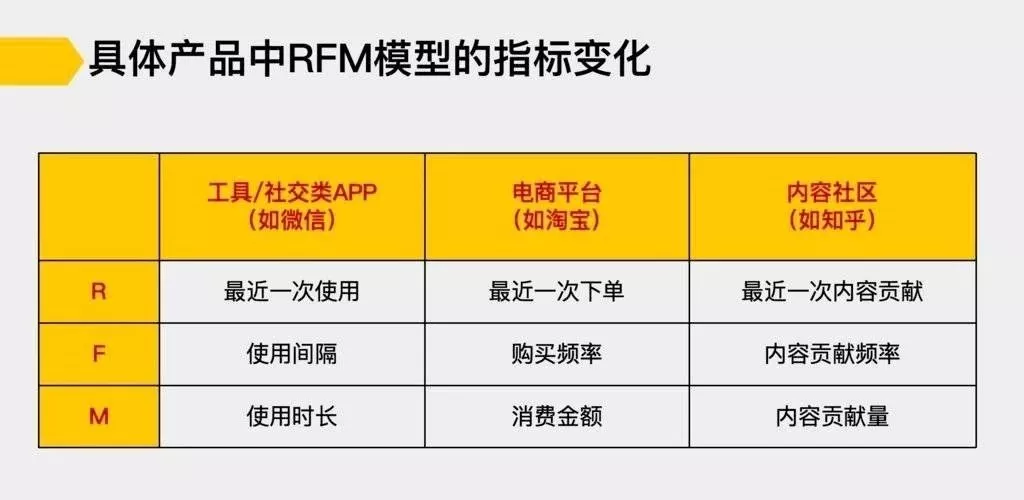R 最近一次交易的时间：R值最大，说明发生交易的时间越久，反之，发生交易的时间越近。

F 交易的频次：F越大，说明交易频繁，是平台的用户用户，反之，用户不够活跃。

M 交易的总金额：M越大，客户价值越高，是平台的重点用户。


通过RFM模型做用户分层，是从所有用户的层面来说的，这种分层方式适合用来制定针对所有用户的运营策略，比如积分、等级、激励等，也可以用来指导在具体的活动中，我们还选择哪些用户，比如电商大促活动中，为了达成销售额你要针对哪些用户呢？

显然是最近有下单、购买频率高、消费金额高的用户了，这是第一优先级的用户，还有就是最近有下单，购买频率低，消费金额高和最近有下单，购买频率高，消费金额低的用户，针对前者，要推荐的是竞品，也就是品质好单价高的商品，针对后者，要推荐的是爆品，也就是销量大价格低的商品。

在制定完整体的策略后，该如何设计后面的细节呢？这里就需要对用户做进一步的分层，比如针对购买频率低、消费金额高的用户，我们可以再次做分层，比如根据购买过的商品品类分层，给买过首饰的用户推荐首饰类的优惠券，给买过衣服的用户推荐衣服类的优惠券，给买过奶粉的用户推荐奶粉的优惠券。

用户分层是一个用户筛选的过程，筛选的颗粒度越细，我们能够制定的策略就越有针对性，那么用户转化的效果就更好。展开全文• 用户活跃、用户价制度分析的在数据分析师的日常工作中经常会碰到，如何根据公司的业务情况对本公司的用户做活跃和价制度的划分是一种常规化的分析工作。 在用户生命周期中，对每个用户进行群体划分，有针对性地做分...

用户活跃、用户价制度分析的在数据分析师的日常工作中经常会碰到，如何根据公司的业务情况对本公司的用户做活跃和价制度的划分是一种常规化的分析工作。

在用户生命周期中，对每个用户进行群体划分，有针对性地做分群分层运营，可以更高地提高营收转化。（用户生命周期指用户从注册账户建立起业务关系到完全终止关系的全过程，它动态地描述了用户在不同阶段的大致特征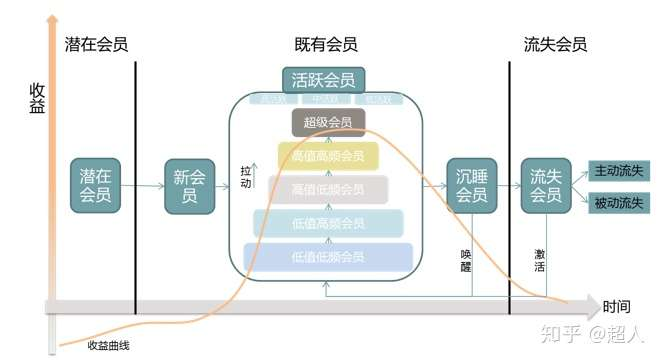近期在开发用户标签的过程中，需要对每个用户打上活跃程度和价值度相关的标签，在经过数据调研，和数据分析师、业务方交流讨论，以及结合之前工作中相关的经验，对这两块内容进行了一次总结。

下面开始说主题：

## 用户活跃度划分

1、维度划分

用户活跃安装一般的口径来说可以划分为：高活跃用户、中活跃用户、低活跃用户、流失用户、现有用户、沉睡用户等常见维度。

首先需要划分用户的流失周期，在流失周期之外的用户为流失用户，在流失周期以内的用户按用户的访问频率再次划分为高、中、低活跃用户。

流失用户、以及高、中、低活跃用户的计算口径是按照用户的访问行为来做划分的，那么现有用户和沉睡用户则是根据用户是否有过购买行为进行划分的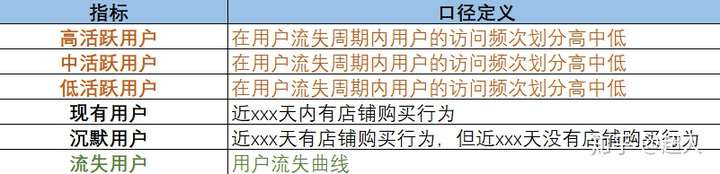2、计算口径

划分高、中、低活跃用户，首先需要定义用户的流失周期。在流失周期以内对用户进行活跃度的划分。

用户流失周期口径：截取某日首次登录的一批用户，观察这批用户在n日后有再次登录行为的用户量及占比（即用户回访率）。为了排除截取日可能会受活动相关因素影响，可以随机截取多个日期用户作为研究对象，取均值计算。

下图中可看出，首日选取的用户在n天后还有回访行为所占的比例在逐日递减。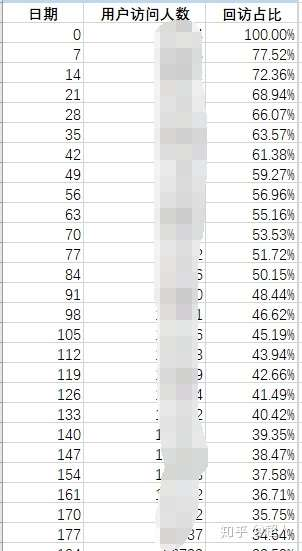根据回访率数值绘制出回访率曲线：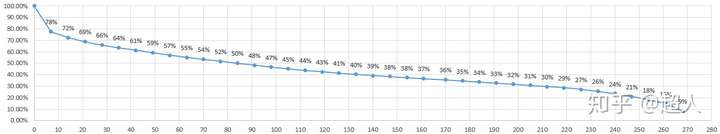可以看出用户在130日以后的回访率低于40%，可认为大部分用户已经流失。因此，可将用户的流失周期划定为130日。

划定用户的流失周期后，在流失周期内根据用户的访问频次（有多少天有过访问行为）及占比，按照二八原则可将用户划分为高、中、低群体。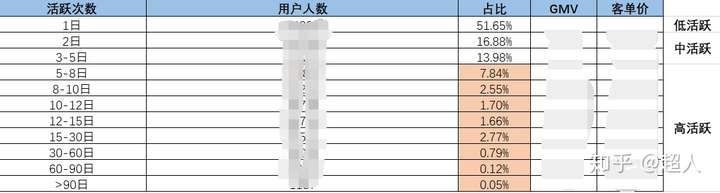关于二八原则我想说的是，原先我认为二八原则是前人拍脑袋定出的一个数字，然而在分析用户价制度的时候，发现占比不到20%的重要价值用户、重要保持用户人群，带来的GMV还真在80%左右。果然古人诚不欺我。这种划分比例还是很有科学依据。

关于现有用户沉默用户的划分原则，是根据用户是否有购买属性来说的，即用户购买后是否有后续的访问行为。可根据用户首次购买后的回访情况来对现有用户和沉默用户做划分。

## 用户价值度划分

1、维度划分

用户价值度按照经典的RFM模型，可以从用户最近一次交易时间、累计交易次数、累计交易金额三个维度出发。对每个维度按照二八原则划分出高价值和低价值用户（高价值占2，低价值占8），对这三个维度中的高、低价值用户通过交叉分析，划分出重要价值用户、重要保持用户、一般价值用户等8个群体。

2、计算口径

① 根据最近一次交易时间分档，按二八原则将用户划分为远、近两个群体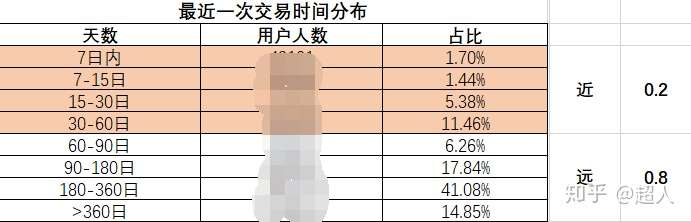② 根据历史累计订单量分档，按二八原则将用户划分为低频次、高频次两个群体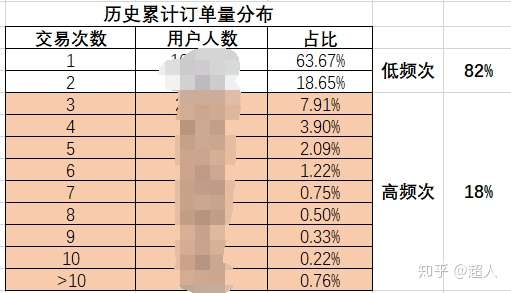③ 根据历史累计交易金额分档，按二八原则将用户划分为低金额、高金额两个群体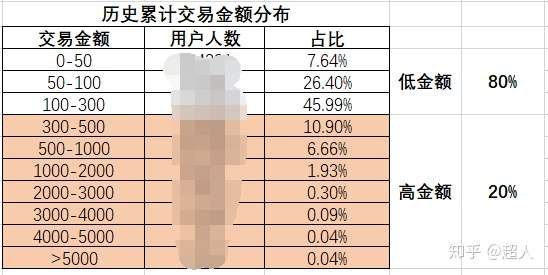最后按照这三个维度对用户分档的原则，将用户划分为8个群体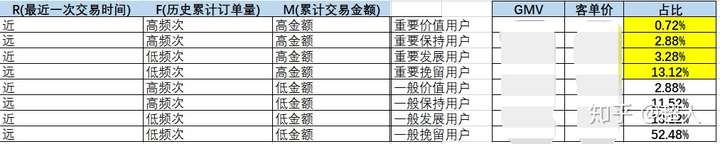标黄的重要用户群体人数占总群体的人数20%左右，但带来的GMV贡献确占到了80%。

根据打标签后的抽样调研情况来看，这种划分方式还是很科学的

展开全文• 核心内容：使用python的sklearn的KMeans算法对电商用户进行分类。 包含内容：数据概览、特征构建、k值选择、模型训练、可视化等。

#### 背景

聚类分析在机器学习领域属于无监督学习的一种，能够根据一些特征对样本数据进行分类。使用聚类分析分完的类具有“类中相似，类间区别”的特点。RFM模型是非常常见的分析用户价值的方法，其核心思想是根据用户的最近购买时间、购买频次、花费金额3个特征来对用户进行分群，针对每个群体采取不同的营销手段。k-means是常用的聚类分析算法之一，基于欧氏距离对样本进行分类。k-means算法运行速度快，能够处理的数据量大，且易于理解。但缺点也很明显，就是算法性能有限，在高维上可能不是最佳选项。在当前动辄上亿的数据量来看，k-means算法是比较好的选择了。还有需要提醒的一点是，一定要结合业务使用算法，任何特征都可能拿来聚类，但是聚类的结果呢，能不能很好的解释和指导业务？如果不能，那么这个算法就没有什么意义。本次使用的数据来源于数据不吹牛公众号，这里也是小打一波广告。
本案例将从一个简单的k-means机器学习模型入手，在介绍聚类算法的同时也简单介绍机器学习的常规步骤。

#### 1、数据概览

首先，我们导入数据，并查看前5行。

import pandas as pd
import numpy as np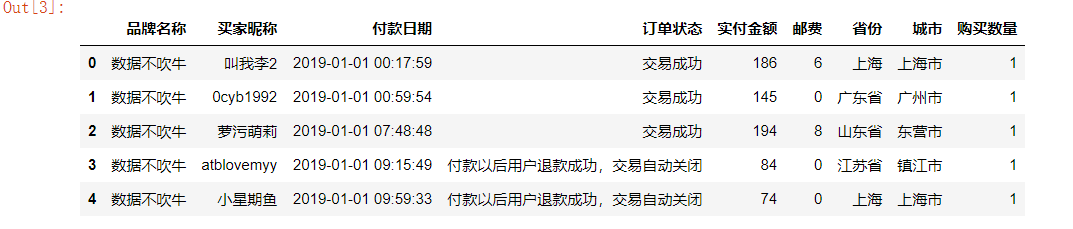总览数据：

df.info()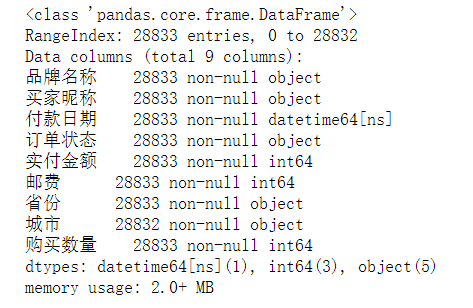数据共有28833行，9列，而且数据非常干净，没有空值，省去了我们处理空值的过程。
根据RFM模型的要求，我们需要的是买家昵称、购买日期、实付金额三个变量。其中购买日期和实付金额用于帮助我们训练模型，买家昵称则让我们确定每个用户所属的类别。

选取交易成功的用户：

df['订单状态'].value_counts()
df = df[df['订单状态'] == '交易成功']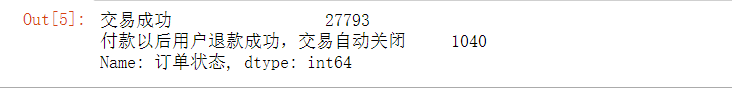选择我们需要的字段：

data = df[['买家昵称', '付款日期',  '实付金额']]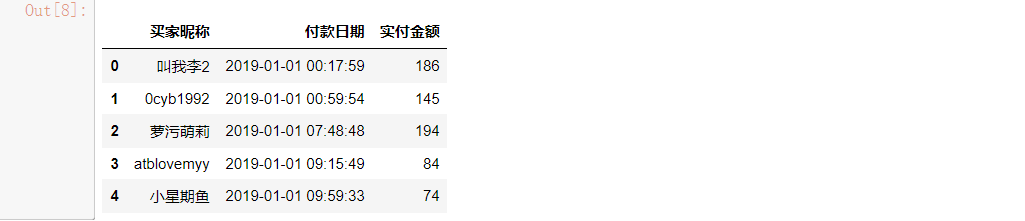有个细节需要注意，订单每一行代表着单个用户的单次购买行为，什么意思呢？如果一个用户在一天内购买了4次，订单表对应记录着4行，而在实际的业务场景中，一个用户在一天内的多次消费行为，应该从整体上看作一次。 ----- 数据不吹牛 小Z

#### 2、特征构建

根据我们的RFM模型，我们需要构建3列数据，这个原始表中是没有直接提供的，需要我们根据原始表的数据来提取。
所以这里需要先提取付款日期数据：

data['paytime'] = pd.to_datetime(data['付款日期'].apply(lambda x:x.date()))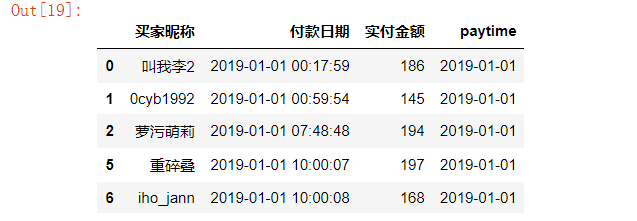根据paytime计算最后一次付款时间距当前的天数（数据引用的背景当前时间是2019-07-01）：

# 提取每个用户最近（最大）的购买日期
data_r = data.groupby('买家昵称')['paytime'].max().reset_index()
# 与当前日期相减，取得最近一次购买距当前的天数。
data_r['recency'] = data_r['paytime'].apply(lambda x:(pd.to_datetime('2019-07-01')-x).days)
# 两个日期相减，得到的数据类型是timedelta类型，要进行数值计算，需要提取出天数数字。
data_r.drop('paytime',axis = 1,inplace = True)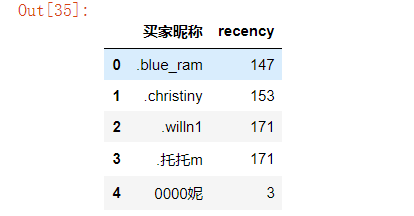提取购买次数数据：

# 分组聚合，得到每个用户发生于不同日期的购买次数
data_f = data.groupby(['买家昵称','paytime'])['付款日期'].count().reset_index()
data_f = data_f.groupby('买家昵称')['paytime'].count().reset_index()
# 修改列名
data_f.rename({'paytime':'frequence'},axis = 1,inplace = True)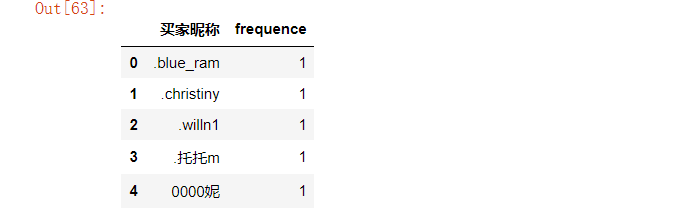提取购买金额数据，这里的金额我们使用每个用户的金额/次：

data_m = data.groupby('买家昵称')['实付金额'].sum().reset_index()
data_m['money'] = data_m['实付金额']/data_f['frequence']
data_m.drop('实付金额',axis = 1,inplace = True)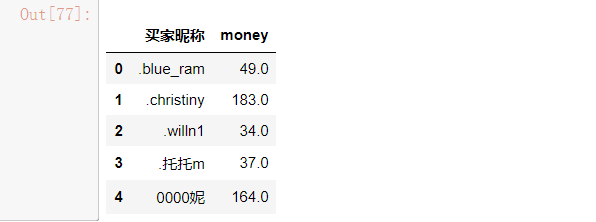所以现在我们已经有了包含recency、frequence、money的3个DataFrame表了，下面合并三个表：

data_rf = pd.merge(data_r,data_f,on = '买家昵称',how = 'inner')
data_rfm = pd.merge(data_rf,data_m, on = '买家昵称',how = 'inner')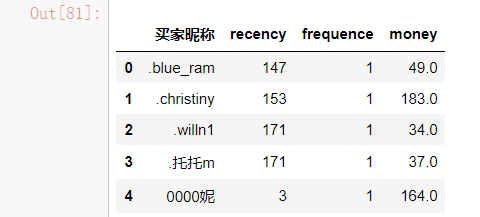#### 3、查看数据分布特征

数据的分布特征会影响算法结果，所以有必要先了解数据的大致分布。

import matplotlib.pyplot as plt
import seaborn as sns
plt.figure(figsize = (6,4))
sns.set(style = 'darkgrid')
sns.countplot(data_rfm['frequence'])sns.distplot(data_rfm['recency'])
plt.title('recency的分布直方图',fontsize = 15)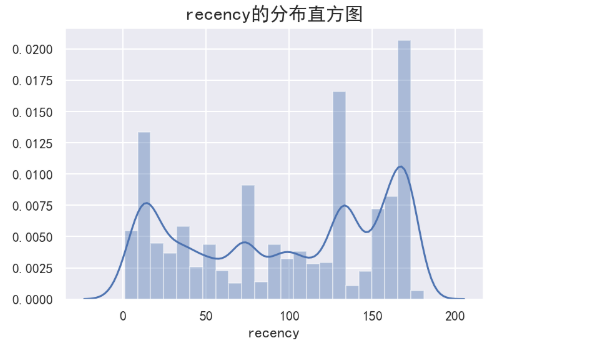sns.distplot(data_rfm['money'],color = 'g')
plt.title('money的分布直方图',fontsize = 15)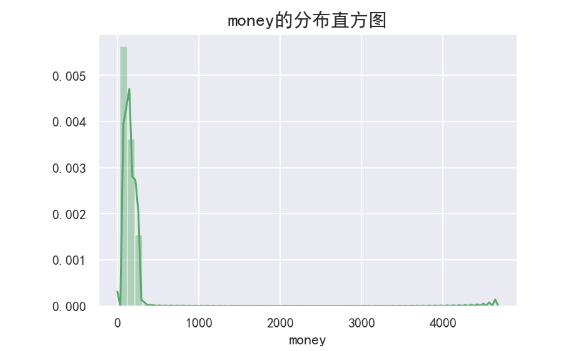#### 4、数据处理及模型构造

首先，对数据进行标准化处理，这是为了消除量纲的影响。

from sklearn import preprocessing
from sklearn.cluster import KMeans
from sklearn import metrics
data_rfm_s = data_rfm.copy()
min_max_scaler = preprocessing.MinMaxScaler()
data_rfm_s = min_max_scaler.fit_transform(data_rfm[['recency','frequence','money']])


K-Means方法有个经常被人诟病的地方，如何选择K值？也就是要把样本分成多少类呢？如果贸然定一个，会太主观，所以还是要用数据说话。一般采用三个指标：

• Calinski-Harabaz Index。通过计算类中各点与类中心的距离平方和来度量类内的紧密度，通过计算各类中心点与数据集中心点距离平方和来度量数据集的分离度，CH指标由分离度与紧密度的比值得到。从而，CH越大代表着类自身越紧密，类与类之间越分散，即更优的聚类结果。
• 轮廓系数。轮廓系数（Silhouette Coefficient），值是介于 [-1,1] ，越趋近于1代表内聚度和分离度都相对较优。也就是尽量选择更大的轮廓系数。
• inertia值。kmeans自带的评价方法，越小说明聚类效果越好。
inertia = []
ch_score = []
ss_score = []
for k in range(2,9):
model = KMeans(n_clusters = k, init = 'k-means++',max_iter = 500)
model.fit(data_rfm_s)
pre = model.predict(data_rfm_s)
ch = metrics.calinski_harabaz_score(data_rfm_s,pre)
ss = metrics.silhouette_score(data_rfm_s,pre)
inertia.append(model.inertia_)
ch_score.append(ch)
ss_score.append(ss)
print(ch_score,ss_score,inertia)


画图可以更加直观看出三个指标的变化：

score = pd.Series([ch_score,ss_score,inertia],index = ['ch_score','ss_score','inertia'])
aa = score.index.tolist()
plt.figure(figsize = (15,6))
j = 1
for i in aa:
plt.subplot(1,3,j)
plt.plot(list(range(2,9)),score[i])
plt.xlabel('k的数目',fontsize = 13)
plt.ylabel(f'{i}值',fontsize = 13)
plt.title(f'{i}值变化趋势',fontsize = 15)
j+=1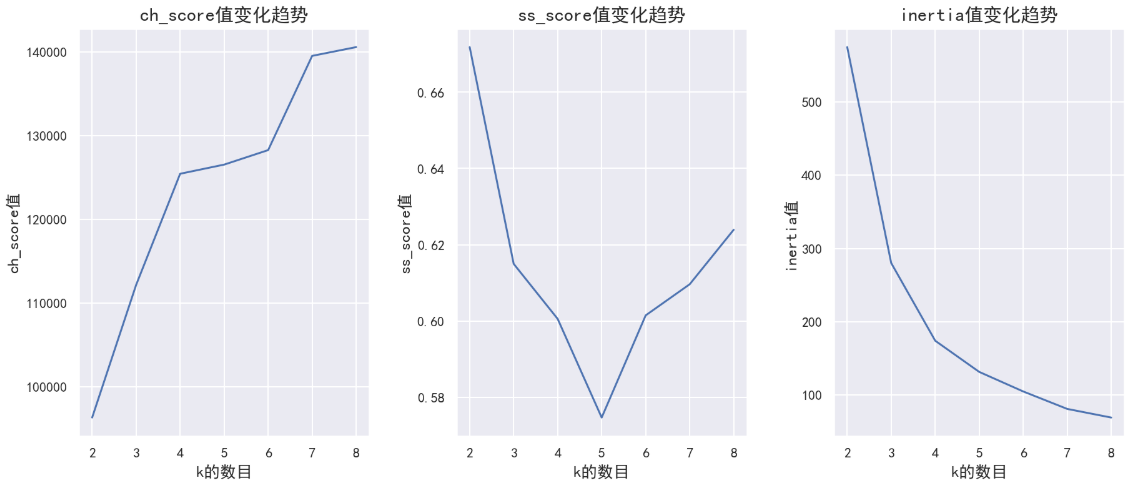根据上图中3个指标综合判断，选择k=4时，各指标变化出现明显的拐点，聚类效果相对较优，所以分成四类比较好。

model = KMeans(n_clusters = 4, init = 'k-means++',max_iter = 500)
model.fit(data_rfm_s)
ppre = model.predict(data_rfm_s)
ppre = pd.DataFrame(ppre)
data = pd.concat([data_rfm,ppre],axis = 1)
data.rename({0:u'cluster'},axis = 1,inplace = True)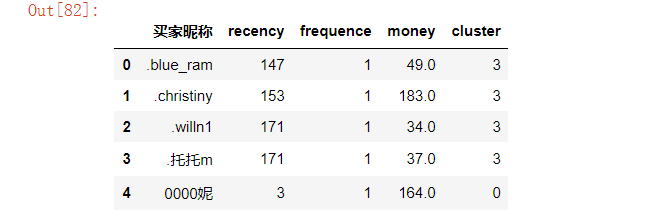可以看出，每个用户都有了对应的类别，并且可以查看每个类别的中心：

labels = model.labels_   # 取得各样本的类别数据
labels = pd.DataFrame(labels,columns = ['类别'])
result = pd.concat([pd.DataFrame(model.cluster_centers_),labels['类别'].value_counts().sort_index()],axis = 1)
result.columns = ['recency','frequence','money','cluster']
result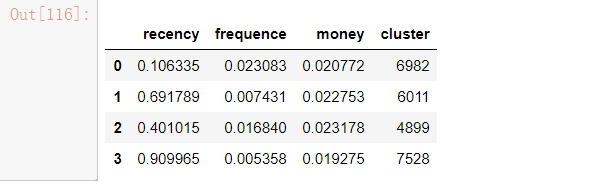cluster=0时的分布状态：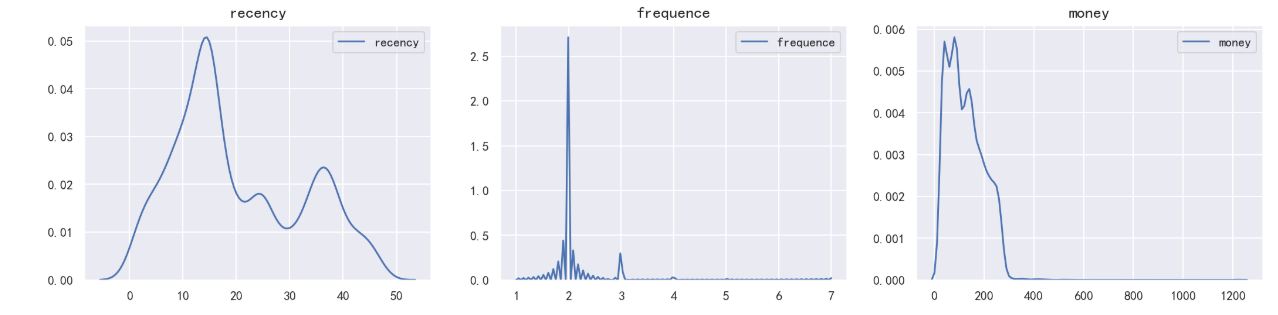cluster=1时的分布状态：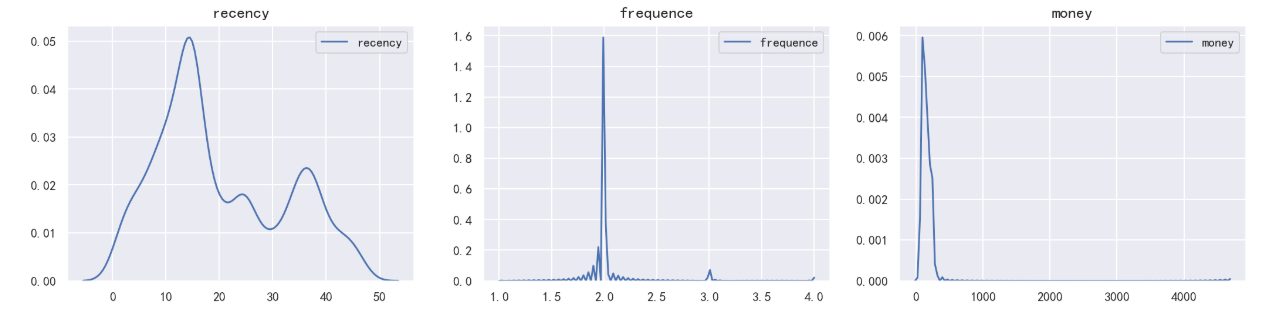cluster=2时的分布状态：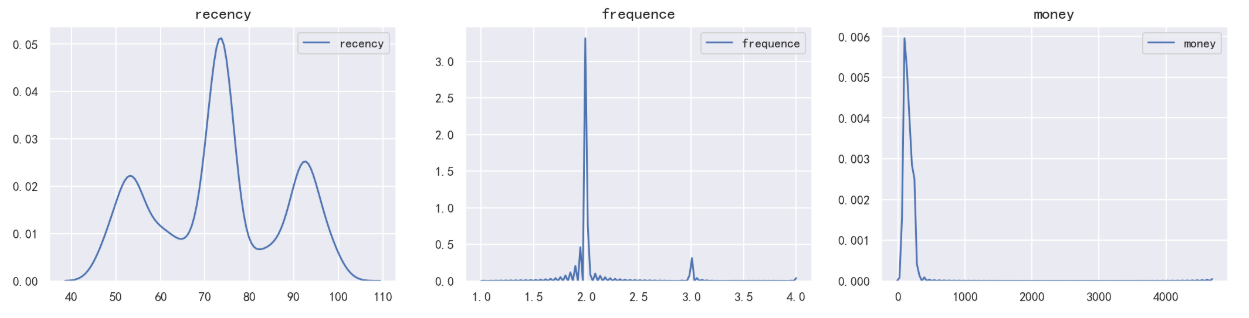cluster=3时的分布状态：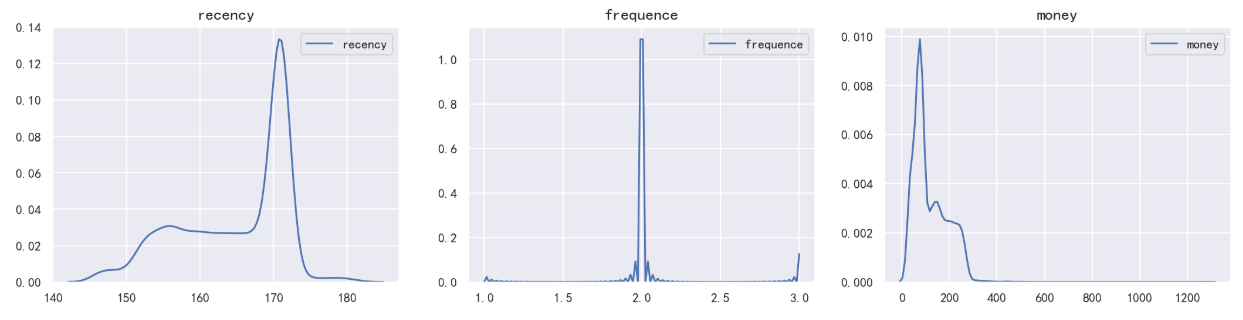展开全文聚类 python 机器学习
• 一套科学的标签体系，能够赋予用户画像以生气，并在用户获取成本飙涨的市场环境下，以最低的成本最大限度地挖掘客户生命周期的价值。随着越来越多的企业重视数据资产在更多业务场景中的应用，标签体系的高效搭建已是...标签系统 数据采集
• 基于RFM模型的用户价值的数据分析报告 分析背景与目的   目前企业的业务逐步从产品为主导转向以客户的需求为主导。一种全新的”以客户为中心“的业务模式正在形成并提升的前所未有的高度。然而与客户保持关系...
• ## 谈谈用户价值

千次阅读 2020-10-27 10:31:00
其实，有些问题，我也没有答案。...1、用户价值定义1.1 用户的商业价值顾名思义，用户在系统中的商业变现能力。这里又分为两种，广告价值，付费价值。很多非业内人士，会以为付费价值显著大于广...大数据 人工智能 算法 java
• 谁在使用我的网站——用户忠诚和价值分析   前面介绍的都是一些用户的行为指标和用户细分，这里要介绍的是基于每个用户行为的综合性的分析和评定，主要包括用户的忠诚度和用户价值。“以用户为中心”的...
• ——毕竟产品是给用户使用的，如果不能提供更好的使用价值用户体验，那么再好的技术也只能是昙花一现。 其次，了解并 分析当前市场中人工智能产品的应用现状 ，从而积累人工智能技术在产品中的技术实现方式与...
• 在10月14日恢复上架后，小红书用不到一个月的时间，把日活跃用户数(DAU)拉回到了7月份的峰值水平。QuestMobile的数据显示，小红书在今年双11期间，DAU再次回到2500万的水位。下架期间，小红书这款明星产品在持续22个...
• 本文向大家介绍Now冥想App如何运用华为分析来挖掘高价值用户，实现付费转化率的逆势增长，希望给同样在增长之路上探索的你带来一点参考。大数据 app
• 文章目录1 概念介绍1.1 概念介绍1.2 关联指标1.3 计算方式1.4 LTV的价值1.5 应用场景1.5.1 宏观方面的几个场景：1.5.2 一个细分的金融场景：1.5.3 预估成本回收期1.5.4 判定渠道质量1.5.5 支持产品运营1.5.6 UE计算2...生命周期 LT
• 首先，我们还得从电商用户LTV（用户生命周期价值）谈起。 在电商运营中，常常会听到用户生命周期价值。为什么要了解用户生命周期价值？ 生命周期指的是一个主体从产生到结束的发展过程。网站用户的生命周期可以理解...
• 定义：LTV值单个用户整个生命周期所产生的价值。 说明：在谈论LTV的计算方法时，容易混淆的点：指标限定的时间范围。LTV的每种计算方法，都一定要明确到底在谈论单批用户的LTV，还是整个游戏所有用户的LTV。 第一...
• ## 客户价值模型：RFM

千次阅读 2021-04-30 08:56:37
比如电商网站要做一次营销活动，需要针对不同价值的客户群体进行分群，对于 高价值用户推荐手表、珠宝等高端商品，对于低价值用户推荐打折促销的廉价商品 ，当然还有以下这些问题都是需要考虑的： 1、谁是最佳...
• APP用户的生命周期价值（LTV）什么是APP用户的生命周期及价值：生命周期是指一个主体从开始到结束的发展过程，比如人生老病死的整个过程。APP用户的生命周期可以解释为用户从与APP建立关系开始到与APP彻底脱离关系的...
• 大家应该很熟悉RFM模型，这是在做用户价值细分常用的方法。主要涵盖的指标有R（Recency）;消费频率（Frequency）;消费金额(Monetary)；RFM模型在用户价值细分上具有很强的解释性和可操作性。但以下为了展示R是如何...
• RFM分析是从周期内最近下单时间R、下单频率F、下单金额平均值M来分析用户价值的一种方法，这个三个维度分别代表了用户的购买的忠诚度、购买粘性、购买力的信息、再结合价格敏感度(本例用户优惠券占比计算）可以...电商运营优化
• 在电商运营中，常常会听到用户生命周期价值。 为什么要了解用户生命周期价值？这要从推广渠道价值说起。 做SaaS的都知道除了CAC（客户获取成本）之外，LTV（用户生命周期价值）也是十分重要的——比如支出2000元营销...电商数据分析 产品运营
• 航空公司客户价值分析 在面向客户制定运营策略、营销策略时，希望能够针对不同的客户推行不同的策略，实现精准化运营，以期获取最大的转化率。客户关系管理是精准化运营的基础，而客户关系管理的核心是客户分类。...python 大数据
• RFM模型是衡量用户价值的重要工具和方法， RFM模型主要由3个 基础指标组成： （1） 最近一次消费（Recency） ， 是指用户上一次购买 时间； （2） 消费频率（Frequency） ， 是指用户在一定时间段内的消费 次数； ...
• RFM模型广泛应用于用户运营之中，是衡量当前用户价值和和客户潜在价值的重要工具和手段。RFM模型主要有如下三个指标： R：Recency —— 客户最近一次交易时间的间隔。R值越大，表示客户交易距今越久，反之则越近； ...RFM模型 R语言 K-means聚类
• import pandas as pd from sklearn.preprocessing import StandardScaler from sklearn.cluster import KMeans data = pd.read_csv(r'credit_card.csv',sep=',',encoding='gbk') print(data.columns) ...sklearn Python数据分析
• RFM分析：是根据用户活跃程度和交易金额贡献，进行客户价值细分的一种方法 一、分析指标 指标 解释 意义 R(Recency)近度 客户最近一次交易时间的间隔  R越小，表示客户越近有交易发生 F...RFM分析
• ## 云计算的概念和价值

万次阅读 多人点赞 2018-05-15 22:28:03
云计算的价值： 资源整合、提高资源利用率 利用虚拟化技术，实现资源的弹性伸缩。 每台服务器虚拟出多台虚拟机，避免原来的服务器只能给某个业务独占的问题。 可通过灵活调整虚拟机的规格（CPU、内存等）...云计算 华为网络技术大赛
• 最近在阅读张良均、王路等人出版的书《python数据分析与挖掘实战》，其中有个案例是介绍航空公司客户价值的分析，其中用到的聚类方法是K-Means方法，我一直把学习的重心放在监督学习上，今天就用这个案例练习一下非...
• 　李开复：创新，我们首先是要看他是不是有创造用户价值，因为一个产品，一个创业公司，他的成败最关键的是看他能不能提供可持续的用户价值。基于这个出发点，你可以有各种方法来提供用户价值，包括商业模式的创新；...电子商务...